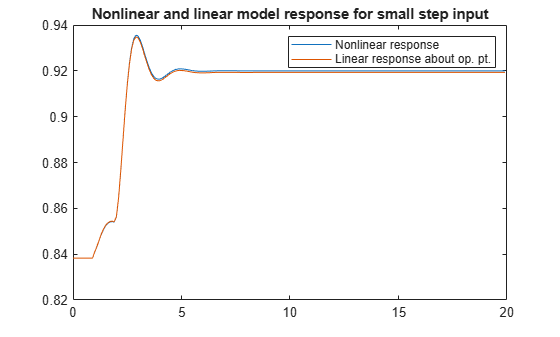# idnlarx/linearize

Linearize nonlinear ARX model

## Syntax

```SYS = linearize(NLSYS,U0,X0) ```

## Description

`SYS = linearize(NLSYS,U0,X0)` linearizes a nonlinear ARX model about the specified operating point `U0` and `X0`. The linearization is based on tangent linearization. For more information about the definition of states for `idnlarx` models, see Definition of idnlarx States.

## Input Arguments

• `NLSYS`: `idnlarx` model.

• `U0`: Matrix containing the constant input values for the model.

• `X0`: Model state values. The states of a nonlinear ARX model are defined by the time-delayed samples of input and output variables. For more information about the states of nonlinear ARX models, see the `getDelayInfo` reference page.

### Note

To estimate `U0` and `X0` from operating point specifications, use the `findop` command.

## Output Arguments

• `SYS` is an `idss` model.

When the Control System Toolbox™ product is installed, `SYS` is an LTI object.

## Examples

collapse all

Linearize a nonlinear ARX model around an operating point corresponding to a simulation snapshot at a specific time.

`load iddata2`

Estimate nonlinear ARX model from sample data.

```nlsys = nlarx(z2,[4 3 10],'tree','custom',... {'sin(y1(t-2)*u1(t))+y1(t-2)*u1(t)+u1(t).*u1(t-13)',... 'y1(t-5)*y1(t-5)*y1(t-1)'},'nlr',[1:5, 7 9]);```

Plot the response of the model for a step input.

`step(nlsys, 20)`The step response is a steady-state value of `0.8383` at `T = 20` seconds.

Compute the operating point corresponding to `T = 20`.

```stepinput = iddata([],[zeros(10,1);ones(200,1)],nlsys.Ts); [x,u] = findop(nlsys,'snapshot',20,stepinput);```

Linearize the model about the operating point corresponding to the model snapshot at `T = 20`.

`sys = linearize(nlsys,u,x);`

Validate the linear model.

Apply a small perturbation `delta_u` to the steady-state input of the nonlinear model `nlsys`. If the linear approximation is accurate, the following should match:

• The response of the nonlinear model `y_nl` to an input that is the sum of the equilibrium level and the perturbation `delta_u` .

• The sum of the response of the linear model to a perturbation input `delta_u` and the output equilibrium level.

Generate a 200-sample perturbation step signal with amplitude 0.1.

`delta_u = [zeros(10,1); 0.1*ones(190,1)];`

For a nonlinear system with a steady-state input of 1 and a steady-state output of 0.8383, compute the steady-state response `y_nl` to the perturbed input `u_nl` . Use equilibrium state values `x` computed previously as initial conditions.

```u_nl = 1 + delta_u; y_nl = sim(nlsys,u_nl,x);```

Compute response of linear model to perturbation input and add it to the output equilibrium level.

`y_lin = 0.8383 + lsim(sys,delta_u);`

Compare the response of nonlinear and linear models.

```time = [0:0.1:19.9]'; plot(time,y_nl,time,y_lin) legend('Nonlinear response','Linear response about op. pt.') title(['Nonlinear and linear model response for small step input'])```## Algorithms

The following equations govern the dynamics of an `idnlarx` model:

`$\begin{array}{l}X\left(t+1\right)=AX\left(t\right)+B\stackrel{˜}{u}\left(t\right)\\ y\left(t\right)=f\left(X,u\right)\end{array}$`

where X(t) is a state vector, u(t) is the input, and y(t) is the output. A and B are constant matrices. $\stackrel{˜}{u}\left(t\right)$ is [y(t), u(t)]T.

The output at the operating point is given by

y* = f(X*, u*)

where X* and u* are the state vector and input at the operating point.

The linear approximation of the model response is as follows:

`$\begin{array}{l}\Delta X\left(t+1\right)=\left(A+{B}_{1}{f}_{X}\right)\Delta X\left(t\right)+\left({B}_{1}{f}_{u}+{B}_{2}\right)\Delta u\left(t\right)\\ \Delta y\left(t\right)={f}_{X}\Delta X\left(t\right)+{f}_{u}\Delta u\left(t\right)\end{array}$`

where

• $\Delta X\left(t\right)=X\left(t\right)-{X}^{*}\left(t\right)$

• $\Delta u\left(t\right)=u\left(t\right)-{u}^{*}\left(t\right)$

• $\Delta y\left(t\right)=y\left(t\right)-{y}^{*}\left(t\right)$

• $B\stackrel{˜}{U}=\left[{B}_{1},{B}_{2}\right]\left[\begin{array}{c}Y\\ U\end{array}\right]={B}_{1}Y+{B}_{2}U$

• ${{f}_{X}=\frac{\partial }{\partial X}f\left(X,U\right)|}_{X*,U*}$

• ${{f}_{U}=\frac{\partial }{\partial U}f\left(X,U\right)|}_{X*,U*}$

### Note

For linear approximations over larger input ranges, use `linapp`.